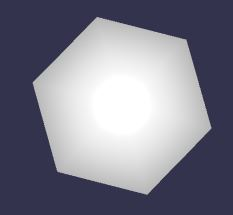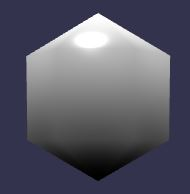## Recommended Posts

The following function minimizes the number of vertices in a mesh.

``````	BABYLON.Mesh.prototype.minimizeVertices = function() {

var _pdata = this.getVerticesData(BABYLON.VertexBuffer.PositionKind);
var _ndata = this.getVerticesData(BABYLON.VertexBuffer.NormalKind);
var _idata = this.getIndices();

var _newPdata = []; //new positions array
var _newIdata =[]; //new indices array

var _mapPtr =0; // new index;
var _uniquePositions = []; // unique vertex positions
for(var _i=0; _i<_idata.length; _i+=3) {
var _facet = [_idata[_i], _idata[_i + 1], _idata[_i+2]]; //facet vertex indices
var _pstring = []; //lists facet vertex positions (x,y,z) as string "xyz""
for(var _j = 0; _j<3; _j++) { //
_pstring[_j] = "";
for(var _k = 0; _k<3; _k++) {
//small values make 0
if (Math.abs(_pdata[3*_facet[_j] + _k]) < 0.0001) {
_pdata[3*_facet[_j] + _k] = 0;
}
_pstring[_j] += _pdata[3*_facet[_j] + _k] + "|";
}
_pstring[_j] = _pstring[_j].slice(0, -1);
}
//check facet vertices to see that none are repeated
// do not process any facet that has a repeated vertex, ie is a line
if(!(_pstring == _pstring || _pstring == _pstring || _pstring == _pstring)) {
//for each facet position check if already listed in uniquePositions
// if not listed add to uniquePositions and set index pointer
// if listed use its index in uniquePositions and new index pointer
for(var _j = 0; _j<3; _j++) {
var _ptr = _uniquePositions.indexOf(_pstring[_j])
if(_ptr < 0) {
_uniquePositions.push(_pstring[_j]);
_ptr = _mapPtr++;
//not listed so add individual x, y, z coordinates to new positions array newPdata
//and add matching normal data to new normals array newNdata
for(var _k = 0; _k<3; _k++) {
_newPdata.push(_pdata[3*_facet[_j] + _k]);
}
}
// add new index pointer to new indices array newIdata
_newIdata.push(_ptr);
}
}
}

_newNdata =[]; //new normal data

BABYLON.VertexData.ComputeNormals(_newPdata, _newIdata, _newNdata);

//create new vertex data object and update
var _vertexData = new BABYLON.VertexData();
_vertexData.positions = _newPdata;
_vertexData.indices = _newIdata;
_vertexData.normals = _newNdata;

_vertexData.applyToMesh(this);

}``````

If you check this playground http://www.babylonjs-playground.com/#1JBMJ3#15  and switch on the Debug Layer you will see the number of vertices is 366.

Comment out line 78 (ie do not apply the minimize vertices function) the number of vertices is 435.

Replacing line 78 with sphere.optimizeIndices() leaves the number of vertices as 435

EDIT

Just to bring to the top RaananW's warning from his post, this will only help if the mesh material is a uniform colour or texture. Textures such as the map of the earth will not seam correctly if the above function is applied to the sphere.

Questions

1. Is this a useful function?
2. Is there anything made not possible by applying the function?
3. Could this already be done with existing functions?

Some Background to The Developement

Playing around with  sphere with a small number of segments (1) (below) I counted 14 vertices but the Debug Layer showed 28The console for the vertex data positions gave Array [ 0, 5, 0, 0, 5, 0, 0, 5, 0, 0, 74 more… ]  and

that for the indices gave Array [ 0, 1, 7, 7, 1, 8, 1, 2, 8, 8, 98 more… ]

This showed two things

(1) vertex positions are repeated, eg  0, 5, 0 is repeated at least three times

(2) The indices for the first facet (one of the triangles used to construct a mesh) 0, 1, 7 gives the vertex positions as (0, 5, 0), (0, 5, 0) and (4.330126941204071, 2.5, 0). Since (0, 5, 0) accounts for two positions the facet 0, 1, 7 is not a true triangle but is a straight line.

So I set about seeing if I could remove the redundancies.

This playground http://www.babylonjs-playground.com/#1JBMJ3#16 shows it was possible.

Next question was did the function affect sub meshes?

This playground http://www.babylonjs-playground.com/#1JBMJ3#13 shows that it does not appear to?

This playground http://www.babylonjs-playground.com/#1JBMJ3#14 (by uncommenting lines 79 and 80) seems to show  that the function will take a flat shaded mesh and reverse it back. Is there a name for a mesh that is not flat shaded? A round shaded mesh does not sound correct.

##### Share on other sites

Hi John,

The babylon vertices format is sometimes redundant, I agree. But minimizing vertices also mean modifying the UV vertex data as well. Try adding a material to the minimzed sphere - http://www.babylonjs-playground.com/#1JBMJ3#17 and you will see how it is changed.

I remember trying to do something similar when I implemented the simplification class. The function is still there, but should be used very carefully. Especially due to this problem with the textures.

So - I think it is helpful, but only when you don't require any material on the mesh.

##### Share on other sites

@RaananW see what you mean, though it depends on the detail in the image used whether the result is acceptable or not. Will continue to experiment and see if there is some way of getting close to the non minimized version.

Am I right in my assumption that the first pair of numbers in uv array are for vertex index 0, next pair for vertex index 1, and so on?

##### Share on other sites

Yep, the uv coordinates in the n index are the two positions in uvs[n*2] and uvs[n*2+1]

so, technically if you find two positions AND uv that are the same, you can minimize them.

##### Share on other sites

@RaananW I think I have got my head around why the need for facets that are in fact lines and hope what I have added to my developing BabylonJS Guide http://babylonjsguide.github.io/advanced/Facets makes sense?

##### Share on other sites

Got a question about the inbetweens -

if you set 3 and 8 to have the same position, the shape of the mesh will eventually change. Wouldn't it?

##### Share on other sites

This is largely what the Blender & I think 3DX Max exporters do.  They also check for the sharing of normals, vertex colors, UV1, UV2, matrix weights, & matrix indexes.  The last 2 are more involved since there is either 4 or 8 per vertex.  Think you should make note that exported meshes will not improve.

One other thing is that multi-materials duplicate the border verts and are sorted within each corresponding sub-mesh.

##### Share on other sites

@RaananW sorry I don't think I have made myself clear. After reading your first answer and looking at some other threads I realised  why my comment about facets just being lines was necessary for some meshes.

On 05/09/2016 at 2:12 PM, JohnK said:

(2) The indices for the first facet (one of the triangles used to construct a mesh) 0, 1, 7 gives the vertex positions as (0, 5, 0), (0, 5, 0) and (4.330126941204071, 2.5, 0). Since (0, 5, 0) accounts for two positions the facet 0, 1, 7 is not a true triangle but is a straight line.

These are needed so that adjacent facets can have different colours. So in the guide what I was trying to say was that these 'line' facets are necessary as a way of allowing a colour change between facets.  With the extra face 3, 7, 9, 8 in place the shape you would get would not be a cube, but as the shape I want is a cube then I obtain this by letting vertices with indices 3 and 8 have the same positions and 7 and 9 have the same positions.

I think, but I may be wrong, that this is the way you would create a shape such as a sphere if you do not want it to be flat shaded.

Not sure whether that has made it better or worse. Still confused let me know.

## Join the conversation

You can post now and register later. If you have an account, sign in now to post with your account.
Note: Your post will require moderator approval before it will be visible.×   Pasted as rich text.   Paste as plain text instead

Only 75 emoji are allowed.